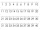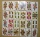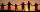# Addition + divisibility - math problems

#### Number of problems found: 9

• 5 digitsYou have the following digits: 9, 8, 0, 1, 5. Write the smallest even five-digit number if one digit is repeated three times and the other digits are not repeated. The digit sum of the number is: a) 9 b) 6 c) 8 d) 23
• What is 16What is the sum of three consecutive even integers such that six more than twice the second is 2/3 of the first increased by 3/2 of the third?
• CalculateCalculate the sum of all three-digit natural numbers divisible by five.
• Sum of odd numbersFind the sum of all odd integers from 13 to 781.
• MultiplesWhat is the sum of the multiples of number 7 that are greater than 30 but less than 56?
• Z9–I–4 MO 2017Numbers 1, 2, 3, 4, 5, 6, 7, 8 and 9 were prepared for a train journey with three wagons. They wanted to sit out so that three numbers were seated in each carriage and the largest of each of the three was equal to the sum of the remaining two. The conduct
• DaughtersThe man conducting the census asks a woman to age of three daughters. Woman says when multiply the age getnumber 72; if their ages add up, get a number of our house, as you see. The man says: That is not enough to calculate their ages. She says: my oldest
• DivisibilityDetermine the smallest integer which divided 11 gives remainder 4 when divided 15 gives remainder 10 and when divided by 19 gives remainder 16.
• DiophantusWe know little about this Greek mathematician from Alexandria, except that he lived around 3rd century A. D. Thanks to an admirer of his, who described his life through an algebraic riddle, we know at least something about his life. Diophantus's youth las

We apologize, but in this category are not a lot of examples.
Do you have an exciting math question or word problem that you can't solve? Ask a question or post a math problem, and we can try to solve it.

We will send a solution to your e-mail address. Solved examples are also published here. Please enter the e-mail correctly and check whether you don't have a full mailbox.

Addition Problems. Divisibility - math problems.